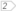#Boost C++ Libraries

...one of the most highly regarded and expertly designed C++ library projects in the world.

This is the documentation for a snapshot of the develop branch, built from commit 8572e0967a.

### Controlling collection types

Freedom of choice
Configuration parameters
Examples

#### Freedom of choice

As has already been said, in STL maps, you can only control the constraints from one of the collections, namely the one that you are viewing. In Boost.Bimap, you can control both and it is as easy as using the STL.The idea is to use the same constraint names that are used in the standard. If you don't specify the collection type, Boost.Bimap assumes that the collection is a set. The instantiation of a bimap with custom collection types looks like this:

```typedef bimap< CollectionType_of<A>, CollectionType_of<B> > bm_type;
```

The following is the list of all supported collection types.

Table 1.2. Collection of Key Types

name

Features

map view type

`set_of`

ordered, unique

`map`

`multiset_of`

ordered

`multimap`

`unordered_set_of`

hashed, unique

`unordered_map`

`unordered_multiset_of`

hashed

`unordered_multimap`

`list_of`

sequenced

`list_map`

`vector_of`

random access

`vector_map`

`unconstrained_set_of`

unconstrained

can not be viewed

`list_of` and `vector_of` map views are not associated with any existing STL associative containers. They are two examples of unsorted associative containers. `unconstrained_set_of` allow the user to ignore a view. This will be explained later.The selection of the collection type affects the possible operations that you can perform with each side of the bimap and the time it takes to do each. If we have:

```typedef bimap< CollectionType_of<A>, CollectionType_of<B> > bm_type;
bm_type bm;
```

The following now describes the resulting map views of the bidirectional map.

• `bm.left` is signature-compatible with LeftMapType`<A,B>`
• `bm.right` is signature-compatible with RightMapType`<B,A>`

#### Configuration parameters

Each collection type template has different parameters to control its behaviour. For example, in `set_of` specification, you can pass a Functor type that compares two types. All of these parameters are exactly the same as those of the standard library container, except for the allocator type. You will learn later how to change the allocator for a bimap.

The following table lists the meanings of each collection type's parameters.

name

`set_of<T,KeyComp>`

`multiset_of<T,KeyComp>`

KeyComp is a Functor that compares two types using a less-than operator. By default, this is `std::less<T>`.

`unordered_set_of<T,HashFunctor,EqualKey>`

`unordered_multiset_of<T,HashFunctor,EqualKey>`

HashFunctor converts a `T` object into an `std::size_t` value. By default it is `boost::hash<T>`.

EqualKey is a Functor that tests two types for equality. By default, the equality operator is `std::equal_to<T>`.

`list_of<T>`

`vector_of<T>`

`unconstrained_set_of<T>`

#### Examples

###### Countries Populations

We want to store countries populations. The requirements are:

1. Get a list of countries in decreasing order of their populations.
2. Given a country, get their population.

Lets create the appropriate bimap.

```typedef bimap<

unordered_set_of< std::string >,
multiset_of< long, std::greater<long> >

> populations_bimap;
```

First of all countries names are unique identifiers, while two countries may have the same population. This is why we choose multi`set_of` for populations.

Using a `multiset_of` for population allow us to iterate over the data. Since listing countries ordered by their names is not a requisite, we can use an `unordered_set_of` that allows constant order look up.

And now lets use it in a complete example

```typedef bimap<

unordered_set_of< std::string >,
multiset_of< long, std::greater<long> >

> population_bimap;

typedef population_bimap::value_type population;

population_bimap pop;
pop.insert( population("China",          1321000000) );
pop.insert( population("India",          1129000000) );
pop.insert( population("United States",   301950000) );
pop.insert( population("Indonesia",       234950000) );
pop.insert( population("Brazil",          186500000) );
pop.insert( population("Pakistan",        163630000) );

std::cout << "Countries by their population:" << std::endl;

// First requirementfor( population_bimap::right_const_iterator
i = pop.right.begin(), iend = pop.right.end();
i != iend ; ++i )
{
std::cout << i->second << " with " << i->first << std::endl;
}

// Second requirementstd::cout << "Population of China: " << pop.left.at("China") << std::endl;
```

 The right map view works like a `std::multimap< long, std::string, std::greater >`, We can iterate over it to print the results in the required order. The left map view works like a `std::unordered_map< std::string, long >`, given the name of the country we can use it to search for the population in constant time
###### Repetitions counter

We want to count the repetitions for each word in a text and print them in order of appearance.

```typedef bimap
<
unordered_set_of< std::string >,
list_of< counter >> word_counter;

typedef boost::tokenizer<boost::char_separator<char> > text_tokenizer;

std::string text=
"Relations between data in the STL are represented with maps."
"A map is a directed relation, by using it you are representing "
"a mapping. In this directed relation, the first type is related to "
"the second type but it is not true that the inverse relationship "
"holds. This is useful in a lot of situations, but there are some "
"relationships that are bidirectional by nature.";

// feed the text into the container
word_counter   wc;
text_tokenizer tok(text,boost::char_separator<char>(" \t\n.,;:!?'\"-"));

for( text_tokenizer::const_iterator it = tok.begin(), it_end = tok.end();
it != it_end ; ++it )
{++ wc.left[*it];
}

// list words with counters by order of appearancefor( word_counter::right_const_iterator
wit = wc.right.begin(), wit_end = wc.right.end();

wit != wit_end; ++wit )
{
std::cout << wit->second << ": " << wit->first;
}
```

 `counter` is an integer that is initialized in zero in the constructor Because the right collection type is `list_of`, the right data is not used a key and can be modified in the same way as with standard maps. When we insert the elements using the left map view, the element is inserted at the end of the list.